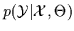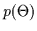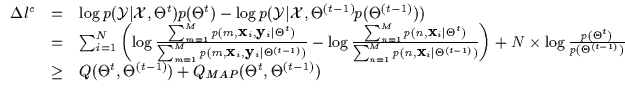Next: Implementation and Interpretation Up: CEM for Gaussian Mixture Previous: Bounding Matrices: The Gate

## MAP Estimation

We have demonstrated a conditional maximum likelihood algorithm for a conditioned mixture of Gaussians. Essentially, this optimized the likelihood of the data given a model. If a prior on the modelis given, we can perform MAP estimation simply by computing a bound on the prior as well. Recall that we had bounded the conditional log-likelihood (as in Equation 7.6) using a Q function. We depict the inclusion of a prior in Equation 7.32. Note how this does not change the derivation of the maximum conditional likelihood solution and merely adds one more bound for the prior to the N bounds computed for each data point for the MLc solution. In fact, the optimization of the prior is identical as it would be for a joint density maximum a posteriori problem (MAP) and the fact that we are optimizing MAPc does not affect it. Thus, a prior on a conditioned mixture model being update with CEM will can be bounded exactly as a prior on a normal mixture model being updated with EM. In theory, it is thus possible to add to the above CEM derivation a variety of priors including non-informative priors, conjugate priors, entropic priors and so on.(7.32)Next: Implementation and Interpretation Up: CEM for Gaussian Mixture Previous: Bounding Matrices: The Gate
Tony Jebara
1999-09-15